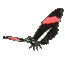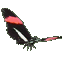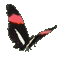Math Help - Line & Parabola Points - Ordered Pairs Graph Game Tips:

-The relation y=ax represents a set of ordered pairs (x,y) forming a straight line through (0,0).
The line y=3x represents points such as (0,0), (1,3), (2,6), (3,9), (4,12), etc.

-The relation y=ax2 represents a set of ordered pairs (x,y) forming a curved line through (0,0).
The curve y=3x2 represents points such as (0,0), (1,3), (2,12), (3,27), (4,48), etc.

- Your Game Score is reduced by the number of butterfly hits.

- To slow the game speed repeat tap/click on the word Slider.
- To increase the game speed repeat tap/click on the word Math.
- Speed can also be adjusted with a keyboard's - and + keys.

- Refresh/Reload the web page to restart the game.